# Electronic Transport and Quantum Phase Transitions of Quantum

• Slides: 37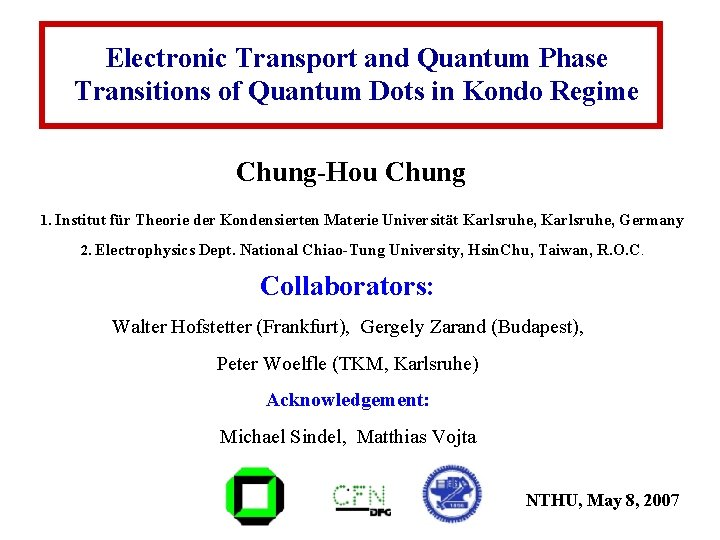Electronic Transport and Quantum Phase Transitions of Quantum Dots in Kondo Regime Chung-Hou Chung 1. Institut für Theorie der Kondensierten Materie Universität Karlsruhe, Germany 2. Electrophysics Dept. National Chiao-Tung University, Hsin. Chu, Taiwan, R. O. C. Collaborators: Walter Hofstetter (Frankfurt), Gergely Zarand (Budapest), Peter Woelfle (TKM, Karlsruhe) Acknowledgement: Michael Sindel, Matthias Vojta NTHU, May 8, 2007Outline • Introduction • Electronic transport and quantum phase transitions in coupled quantum dots: Model (I): parallel coupled quantum dots, 2 -channel Kondo, non-trivial quantum critical point Model (II): side-coupled quantum dots, 1 -channel Kondo, Kosterlitz-Thouless quantum transition • Conclusions and Outlook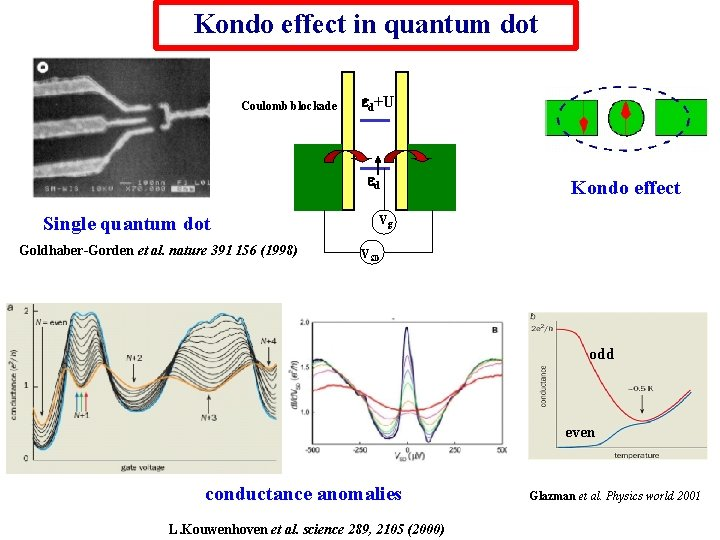Kondo effect in quantum dot Coulomb blockade ed+U ed Single quantum dot Goldhaber-Gorden et al. nature 391 156 (1998) Kondo effect Vg VSD odd even conductance anomalies L. Kouwenhoven et al. science 289, 2105 (2000) Glazman et al. Physics world 2001Kondo effect in metals with magnetic impurities (Kondo, 1964) log. T electron-impurity scattering via spin exchange coupling (Glazman et al. Physics world 2001) At low T, spin-flip scattering off impurities enhances Ground state is spin-singlet Resistance increases as T is loweredKondo effect in quantum dot (J. von Delft)Kondo effect in quantum dotKondo effect in quantum dot Anderson Model local energy level : d ∝ Vg charging energy : U level width : All tunable! Γ= 2πV 2ρ New energy scale: Tk ≈ Dexp(-p. U/ G) For T < Tk : Impurity spin is screened (Kondo screening) d Spin-singlet ground state Local density of states developes Kondo resonanceKondo Resonance of a single quantum dot Spectral density at T=0 M. Sindel particle-hole symmetry Universal scaling of T/Tk L. Kouwenhoven et al. science 2000 P-H symmetry phase shift Fredel sum rule = p/2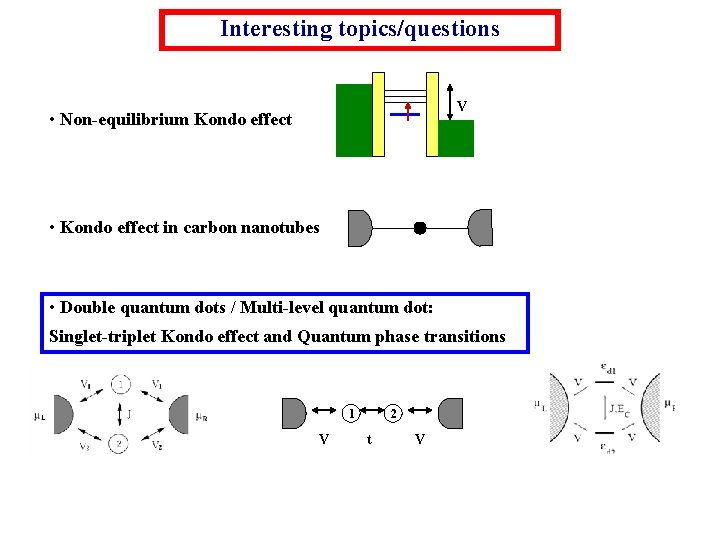Interesting topics/questions V • Non-equilibrium Kondo effect • Kondo effect in carbon nanotubes • Double quantum dots / Multi-level quantum dot: Singlet-triplet Kondo effect and Quantum phase transitions 1 V 2 t V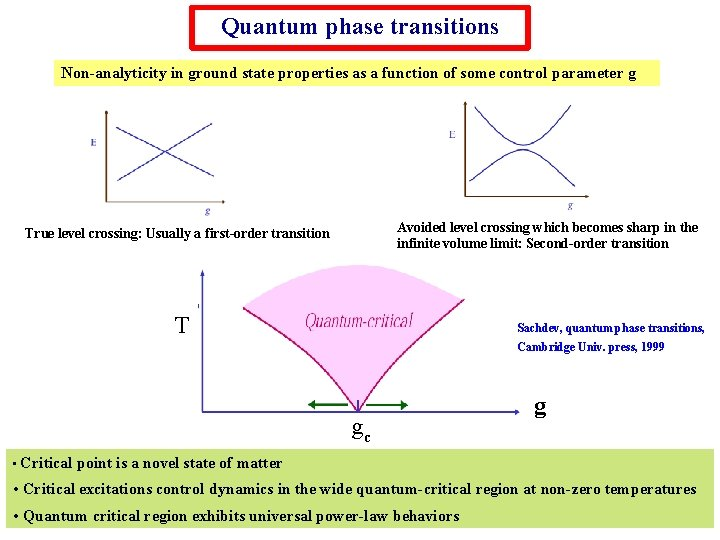Quantum phase transitions Non-analyticity in ground state properties as a function of some control parameter g Avoided level crossing which becomes sharp in the infinite volume limit: Second-order transition True level crossing: Usually a first-order transition T Sachdev, quantum phase transitions, Cambridge Univ. press, 1999 gc • Critical g point is a novel state of matter • Critical excitations control dynamics in the wide quantum-critical region at non-zero temperatures • Quantum critical region exhibits universal power-law behaviorsRecent experiments on coupled quantum dots (I). C. M. Macrus et al. Science, 304, 565 (2004) Two quantum dots coupled through an open conducting region which mediates an antiferromagnetic spin-spin coupling • • For odd number of electrons on both dots, splitting of zero bias Kondo resonance is observed for strong spin exchange coupling.(II). Von der Zant et al. cond-mat/0508395, (PRL, 2005) • A quantum dot coupled to magnetic impurities in the leads • Antiferromagnetic spin coupling between impurity and dot suppresses Kondo effect • Kondo peak restored at finite temperatures and magnetic fields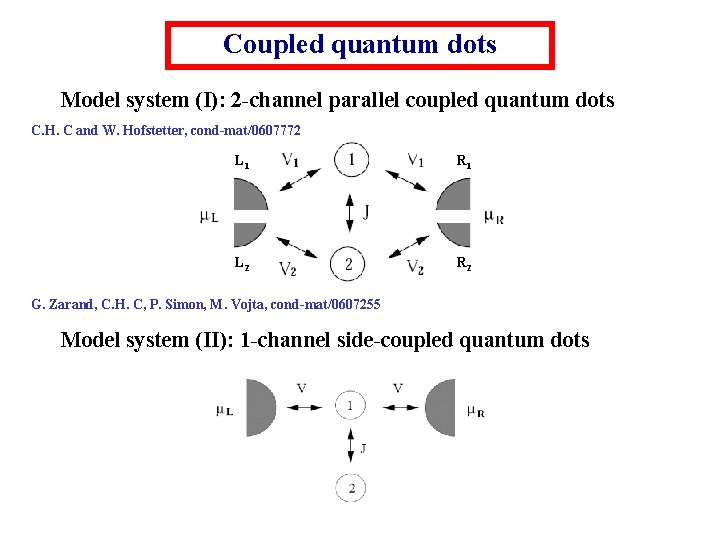Coupled quantum dots Model system (I): 2 -channel parallel coupled quantum dots C. H. C and W. Hofstetter, cond-mat/0607772 L 1 R 1 L 2 R 2 G. Zarand, C. H. C, P. Simon, M. Vojta, cond-mat/0607255 Model system (II): 1 -channel side-coupled quantum dotsNumerical Renormalization Group (NRG) K. G. Wilson, Rev. Mod. Phys. 47, 773 (1975) W. Hofstetter, Advances in solid state physics 41, 27 (2001) Non-perturbative numerical method by Wilson to treat quantum impurity problem Logarithmic discretization of the conduction band Anderson impurity model is mapped onto a linear chain of fermions Iteratively diagonalize the chain and keep low energy levels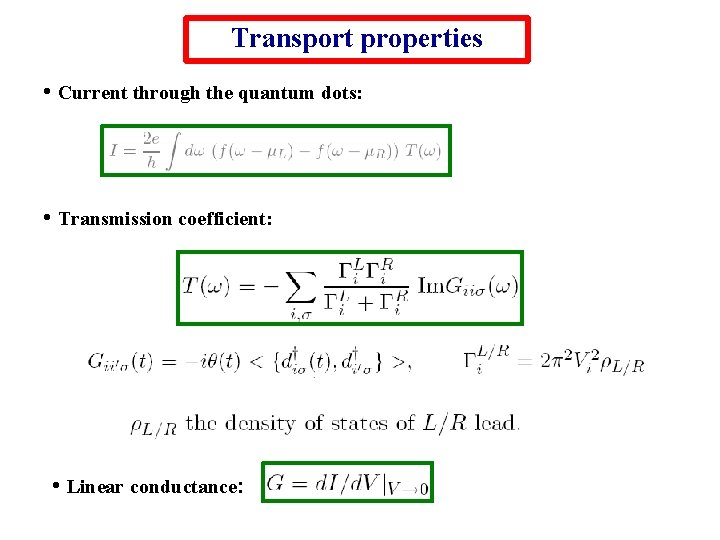Transport properties • Current through the quantum dots: • Transmission coefficient: • Linear conductance: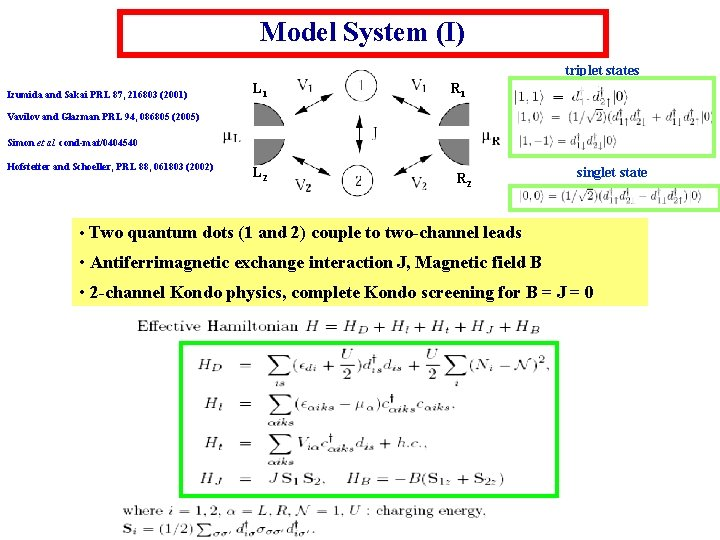Model System (I) triplet states Izumida and Sakai PRL 87, 216803 (2001) L 1 R 1 Vavilov and Glazman PRL 94, 086805 (2005) Simon et al. cond-mat/0404540 Hofstetter and Schoeller, PRL 88, 061803 (2002) L 2 R 2 singlet state • Two quantum dots (1 and 2) couple to two-channel leads • Antiferrimagnetic exchange interaction J, Magnetic field B • 2 -channel Kondo physics, complete Kondo screening for B = J = 0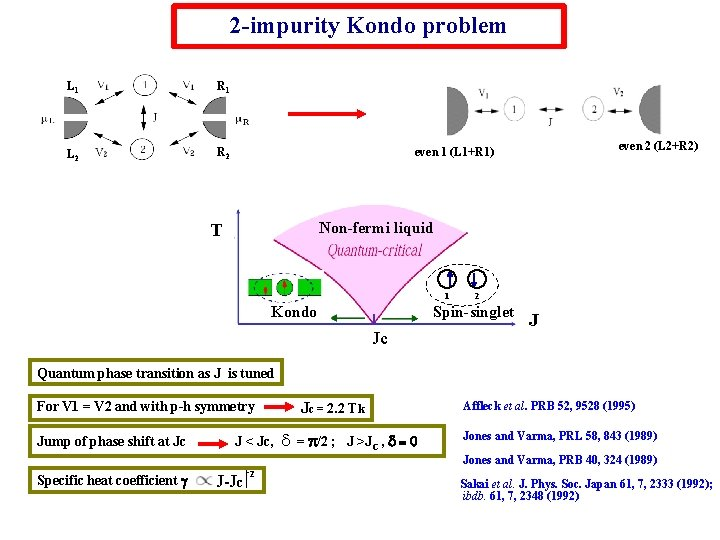2 -impurity Kondo problem L 1 R 1 L 2 R 2 even 2 (L 2+R 2) even 1 (L 1+R 1) Non-fermi liquid T 1 Kondo 2 Spin-singlet Jc J Quantum phase transition as J is tuned For V 1 = V 2 and with p-h symmetry Jump of phase shift at Jc Jc = 2. 2 Tk J < Jc, d = p/2 ; J >JC , d = 0 Affleck et al. PRB 52, 9528 (1995) Jones and Varma, PRL 58, 843 (1989) Jones and Varma, PRB 40, 324 (1989) Specific heat coefficient g J-Jc -2 Sakai et al. J. Phys. Soc. Japan 61, 7, 2333 (1992); ibdb. 61, 7, 2348 (1992)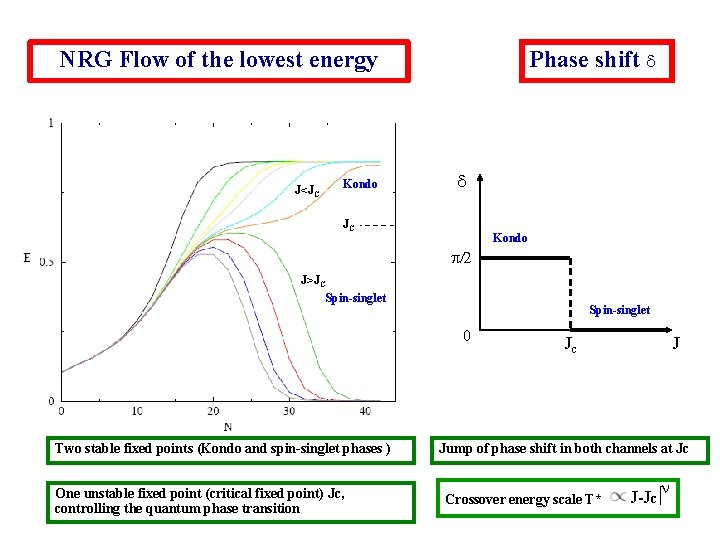NRG Flow of the lowest energy J<JC Kondo Phase shift d d JC Kondo p/2 J>JC Spin-singlet 0 Two stable fixed points (Kondo and spin-singlet phases ) One unstable fixed point (critical fixed point) Jc, controlling the quantum phase transition Jc J Jump of phase shift in both channels at Jc Crossover energy scale T* J-Jc n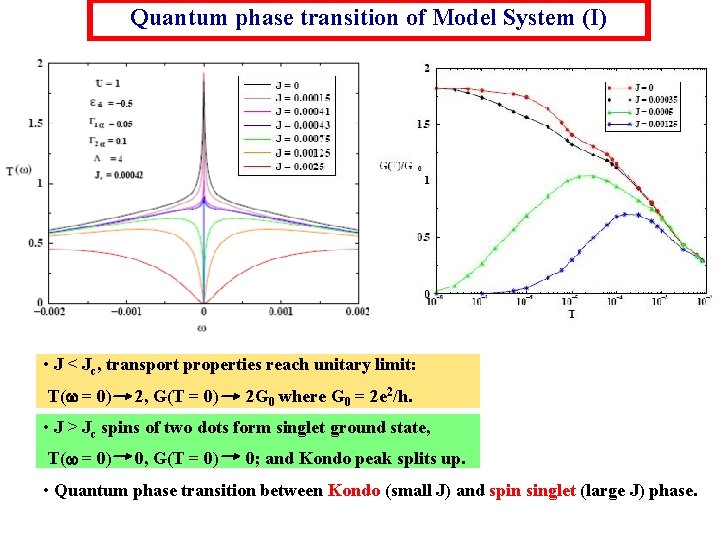Quantum phase transition of Model System (I) • J < Jc, transport properties reach unitary limit: T( = 0) 2, G(T = 0) 2 G 0 where G 0 = 2 e 2/h. • J > Jc spins of two dots form singlet ground state, T( = 0) 0, G(T = 0) 0; and Kondo peak splits up. • Quantum phase transition between Kondo (small J) and spin singlet (large J) phase.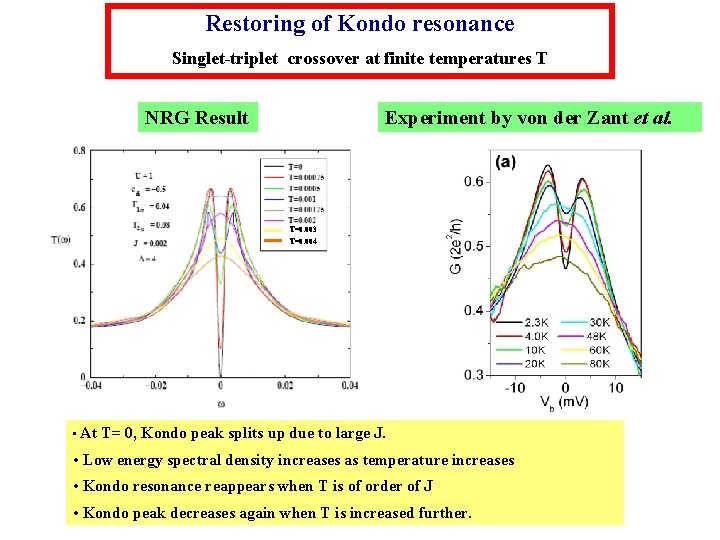Restoring of Kondo resonance Singlet-triplet crossover at finite temperatures T NRG Result Experiment by von der Zant et al. T=0. 003 T=0. 004 • At T= 0, Kondo peak splits up due to large J. • Low energy spectral density increases as temperature increases • Kondo resonance reappears when T is of order of J • Kondo peak decreases again when T is increased further.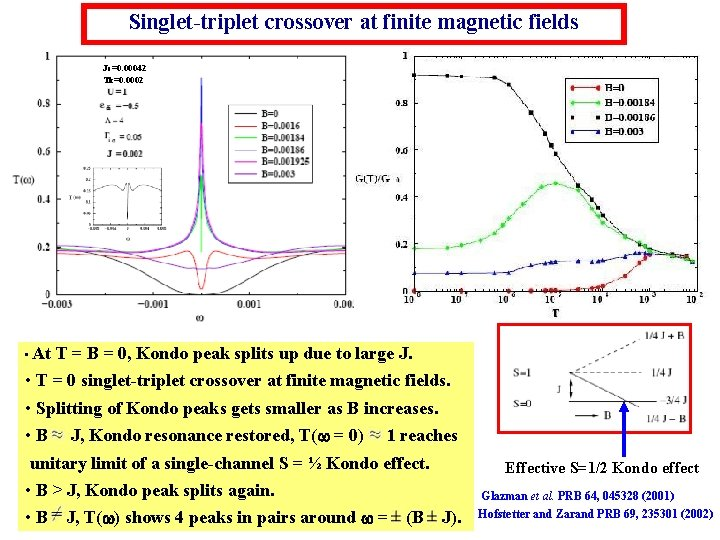Singlet-triplet crossover at finite magnetic fields Jc=0. 00042 Tk=0. 0002 • At T = B = 0, Kondo peak splits up due to large J. • T = 0 singlet-triplet crossover at finite magnetic fields. • Splitting of Kondo peaks gets smaller as B increases. • B J, Kondo resonance restored, T( = 0) 1 reaches unitary limit of a single-channel S = ½ Kondo effect. • B > J, Kondo peak splits again. • B J, T( ) shows 4 peaks in pairs around = (B J). Effective S=1/2 Kondo effect Glazman et al. PRB 64, 045328 (2001) Hofstetter and Zarand PRB 69, 235301 (2002)Singlet-triplet crossover at finite field and temperature J=0. 007, Jc=0. 005, Tk=0. 0025, T=0. 00001, in step of 400 B B in Step of 0. 001 J=-0. 005, Tk=0. 0025 NRG: P-h symmetry EXP: P-h asymmetry Antiferromagnetic J>0 J close to Jc, smooth crossover J >> Jc, sharper crossover Ferromagnetic J<0 splitting of Kondo peak due to Zeemann splitting of up and down spins splitting is linearly proportional to B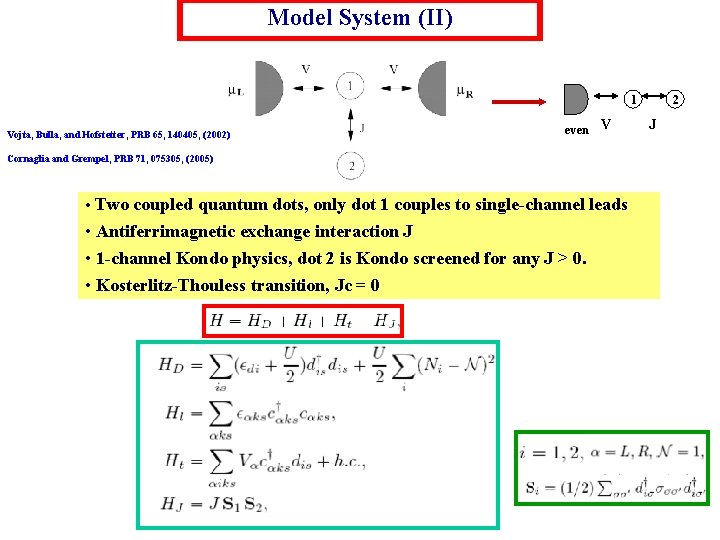Model System (II) 1 Vojta, Bulla, and Hofstetter, PRB 65, 140405, (2002) even V Cornaglia and Grempel, PRB 71, 075305, (2005) • Two coupled quantum dots, only dot 1 couples to single-channel leads • Antiferrimagnetic exchange interaction J • 1 -channel Kondo physics, dot 2 is Kondo screened for any J > 0. • Kosterlitz-Thouless transition, Jc = 0 2 J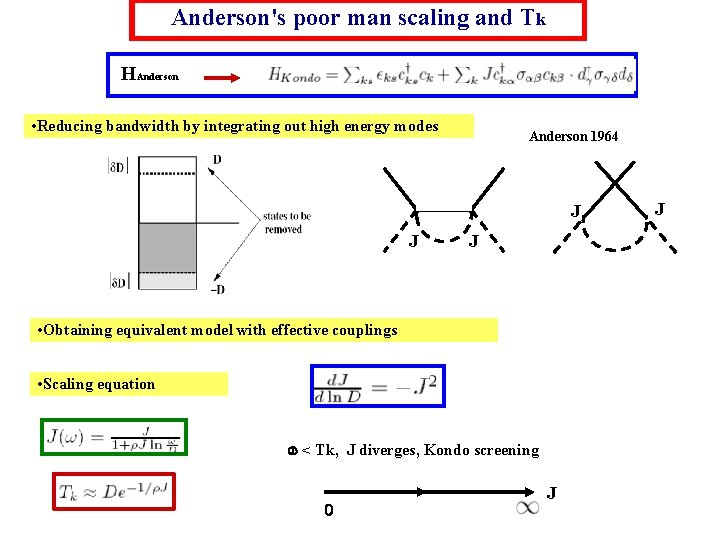Anderson's poor man scaling and Tk HAnderson • Reducing bandwidth by integrating out high energy modes Anderson 1964 J J J • Obtaining equivalent model with effective couplings • Scaling equation < Tk, J diverges, Kondo screening 0 J J2 stage Kondo effect 1 st stage Kondo screening Jk: Kondo coupling Jk 4 V 2/U 2 nd stage Kondo screening D rc Tk J: AF coupling btw dot 1 and 2 1/G dip in DOS of dot 1NRG: Spectral density of Model (II) U=1 Log (T*) ed=-0. 5 G=0. 1 Tk=0. 006 L=2 1/J Kosterlitz-Thouless quantum transition Kondo J 8 0 No 3 rd unstable fixed point corresponding to the critical point spin-singlet Crossover energy scale T* exponentially depends on |J-Jc|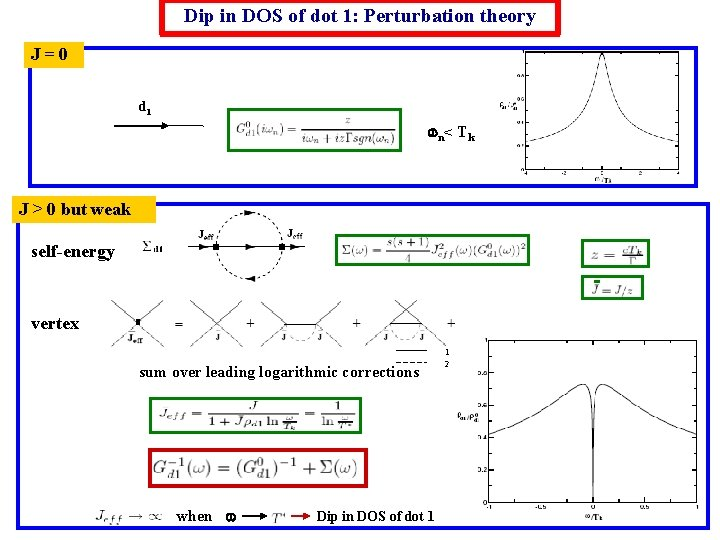Dip in DOS of dot 1: Perturbation theory J=0 d 1 n < T k J > 0 but weak self-energy vertex sum over leading logarithmic corrections when Dip in DOS of dot 1 1 2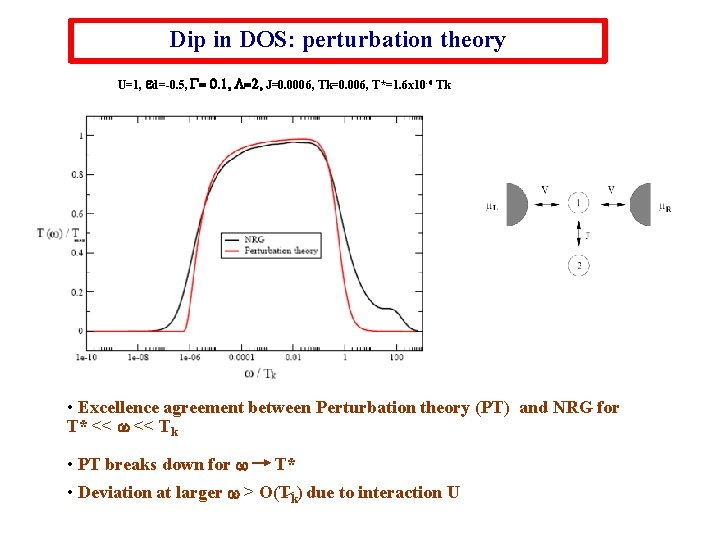Dip in DOS: perturbation theory U=1, ed=-0. 5, G= 0. 1, L=2, J=0. 0006, Tk=0. 006, T*=1. 6 x 10 -6 Tk • Excellence agreement between Perturbation theory (PT) and NRG for T* << Tk • PT breaks down for T* • Deviation at larger > O(Tk) due to interaction U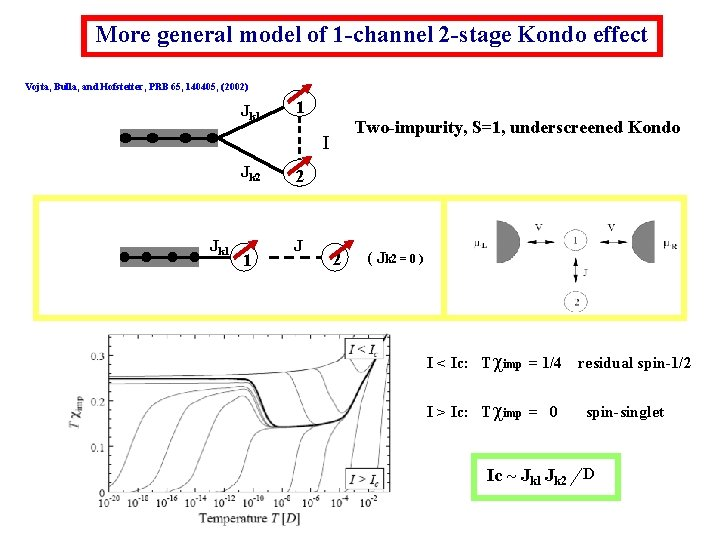More general model of 1 -channel 2 -stage Kondo effect Vojta, Bulla, and Hofstetter, PRB 65, 140405, (2002) Jk 1 1 Two-impurity, S=1, underscreened Kondo I Jk 2 Jk 1 1 2 J 2 ( Jk 2 = 0 ) I < Ic: Tcimp = 1/4 I > Ic: Tcimp = 0 residual spin-1/2 spin-singlet Ic ~ Jk 1 Jk 2 DOptical conductivity J 1 U=1 Dot 2 ed=-0. 5 G=0. 1 Sindel, Hofstetter, von Delft, Kindermann, PRL 94, 196602 (2005) Linear AC conductivity Tk=0. 006 ‘ ‘ L=2Comparison between two models Model (I) 2 impurity, S=1, Two-channel Kondo L 1 R 1 L 2 R 2 Model (II) 2 impurity, S=1, One-channel Kondo Jk 1 J Jk 2 complete Kondo screening quantum critical point J underscreened Kondo K-T transition spin-singlet J-Jc n 0 Kondo 8 Kondo T* 2 8 x Jc 2 1 even 2 (L 2+R 2) even 1 (L 1+R 1) 0 J J spin-singlet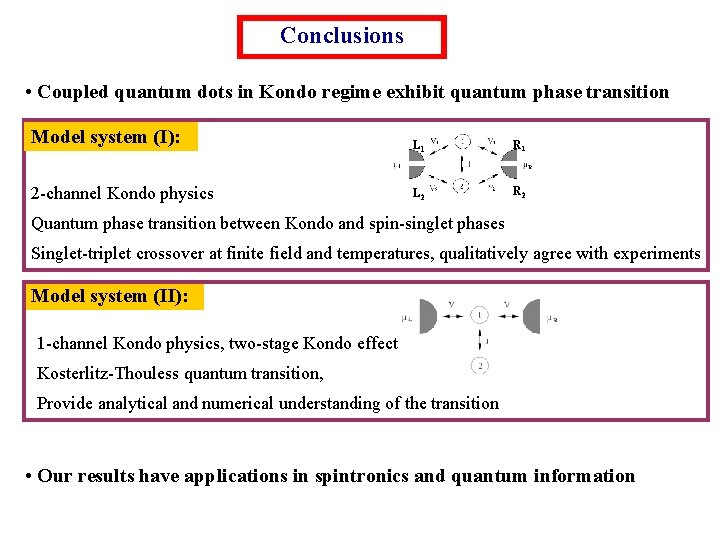Conclusions • Coupled quantum dots in Kondo regime exhibit quantum phase transition Model system (I): 2 -channel Kondo physics L 1 R 1 L 2 R 2 Quantum phase transition between Kondo and spin-singlet phases Singlet-triplet crossover at finite field and temperatures, qualitatively agree with experiments Model system (II): 1 -channel Kondo physics, two-stage Kondo effect Kosterlitz-Thouless quantum transition, Provide analytical and numerical understanding of the transition • Our results have applications in spintronics and quantum information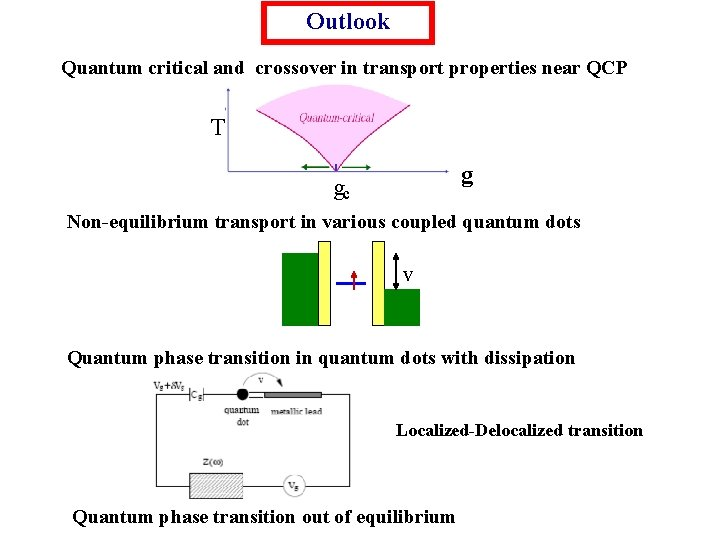Outlook Quantum critical and crossover in transport properties near QCP T g gc Non-equilibrium transport in various coupled quantum dots V Quantum phase transition in quantum dots with dissipation Localized-Delocalized transition Quantum phase transition out of equilibrium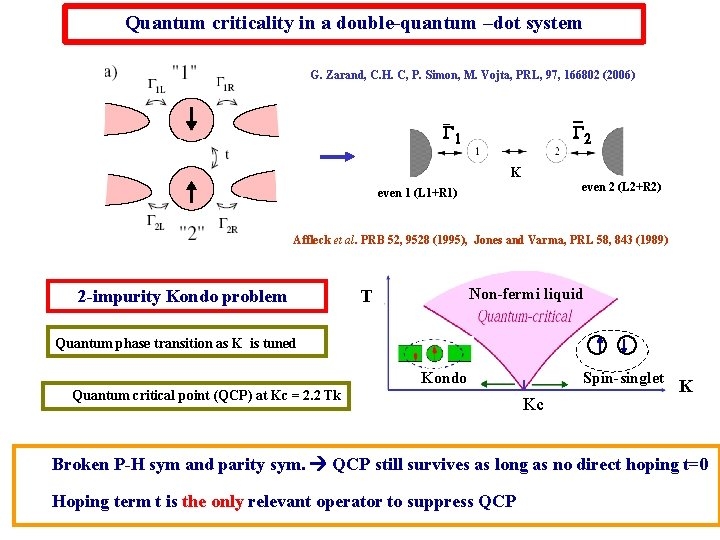Quantum criticality in a double-quantum –dot system G. Zarand, C. H. C, P. Simon, M. Vojta, PRL, 97, 166802 (2006) _ G 2 _ G 1 K even 2 (L 2+R 2) even 1 (L 1+R 1) Affleck et al. PRB 52, 9528 (1995), Jones and Varma, PRL 58, 843 (1989) 2 -impurity Kondo problem Non-fermi liquid T Quantum phase transition as K is tuned Kondo Quantum critical point (QCP) at Kc = 2. 2 Tk Spin-singlet Kc K Broken P-H sym and parity sym. QCP still survives as long as no direct hoping t=0 Hoping term t is the only relevant operator to suppress QCP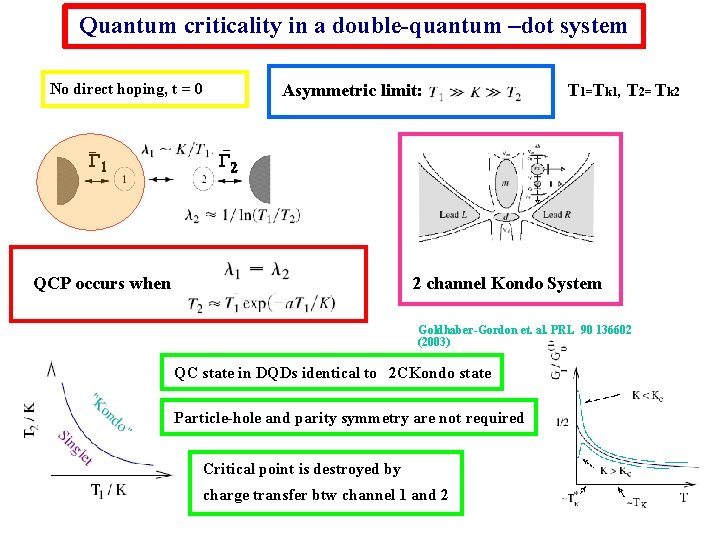Quantum criticality in a double-quantum –dot system Asymmetric limit: No direct hoping, t = 0 _ T 1=Tk 1, T 2= Tk 2 _2 G G 1 K QCP occurs when 2 channel Kondo System Goldhaber-Gordon et. al. PRL 90 136602 (2003) QC state in DQDs identical to 2 CKondo state Particle-hole and parity symmetry are not required Critical point is destroyed by charge transfer btw channel 1 and 2Transport of double-quantum-dot near QCP No direct hoping, t=0 Finite hoping t QCP survives without P-H and parity symmetry QCP is destroyed, smooth crossover << suppress hoping effect observe QCP in QD Exp. C. M. Macrus et al. Science, 304, 565 (2004)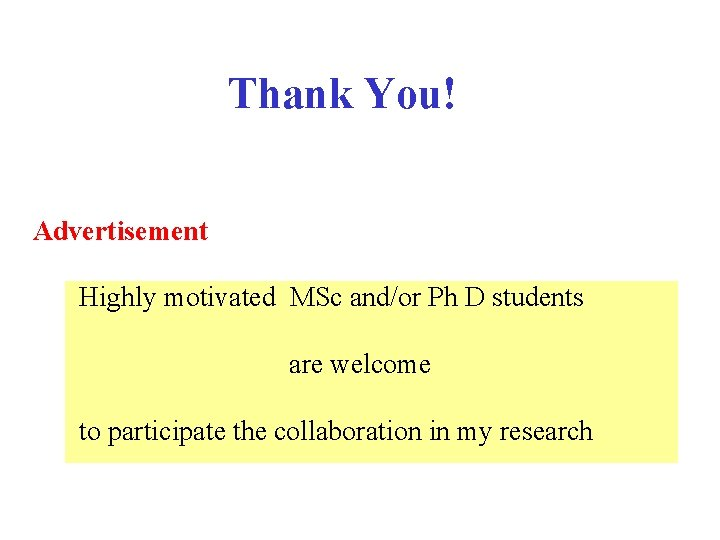Thank You! Advertisement Highly motivated MSc and/or Ph D students are welcome to participate the collaboration in my research